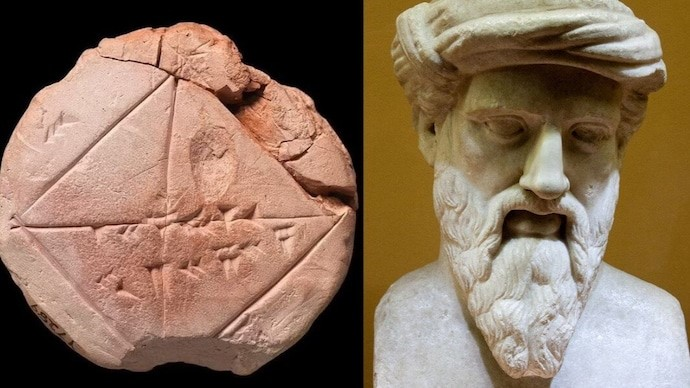# PYTHAGORAS

9th October, 2023 Science and TechnologyContext

While the Greek philosopher Pythagoras, born in 570 BC, has long been accredited with creating the mathematical concept that determines the missing side of a right-angled triangle, fresh evidence appears to debunk this.

Details

• Top of FormPythagoras, a Greek mathematician and philosopher, is best known for the Pythagorean Theorem, a fundamental concept in geometry.
• This theorem has profound applications in mathematics and science and has left an indelible mark on the field of mathematics.
• The Pythagorean Theorem, a fundamental concept in geometry attributed to the Greek philosopher Pythagoras, has long been a cornerstone of mathematical education.
• However, recent findings have cast doubt on the conventional narrative surrounding this theorem. An ancient Babylonian tablet, known as YBC 7289, dating back to 1800-1600 BC, suggests that the Pythagorean Theorem may have predated Pythagoras by over a millennium.
• This revelation challenges the established historical account and raises questions about the origin and dissemination of mathematical knowledge.

The Babylonian Discovery:

• Mathematician Bruce Ratner, with a background in Mathematical Statistics and Probability, uncovered this groundbreaking revelation.
• YBC 7289, a clay tablet from ancient Babylon, played a pivotal role in this discovery. It contains mathematical principles related to the Pythagorean Theorem.
• Ratner's examination of the tablet revealed that the Babylonians employed the Pythagorean Theorem to calculate the length of a rectangle's diagonal, demonstrating its existence long before Pythagoras.Pythagoras: Popularizer, Not Pioneer:

• While Pythagoras is traditionally credited with the Pythagorean Theorem, the Babylonian tablet suggests that he might not have been its originator.
• The theorem's existence in Babylonian mathematics predates Pythagoras's birth by centuries, challenging the idea of him being its pioneer.
• It is possible that Pythagoras encountered the theorem through oral tradition and played a role in its popularization.

Babylonian Understanding of Mathematics:

• Ratner's research highlights that Babylonian mathematicians possessed a deep understanding of the Pythagorean Theorem and other advanced mathematical concepts.
• Their use of a base-60 counting system allowed them to work with fractions and irrational numbers, including the square root of two, a key component of the theorem.
• This newfound knowledge reveals the depth of mathematical understanding in ancient Mesopotamia.

Implications for Mathematical History:

• The discovery of the Babylonian tablet challenges conventional wisdom about the theorem's origin and the scarcity of original Pythagorean sources.
• It underscores the role of Pythagoras as a popularizer of mathematical knowledge rather than its original creator.
• This revelation prompts a reevaluation of the inheritance and transmission of mathematical ideas in antiquity.

Pythagoras - The Man

• Pythagoras was born around 570 BCE on the Greek island of Samos and is considered one of the earliest mathematicians and philosophers.
• He founded a philosophical and religious movement known as Pythagoreanism, which emphasized mathematics, music, and the pursuit of knowledge.
• Pythagoras is often credited with coining the term "mathematics" and made significant contributions to various fields, including geometry, number theory, and music theory.

The Pythagorean Theorem

• The Pythagorean Theorem is a fundamental principle in geometry that relates to right-angled triangles.
• It states that in a right triangle, the square of the length of the hypotenuse (the side opposite the right angle) is equal to the sum of the squares of the lengths of the other two sides. Mathematically, it can be expressed as: a² + b² = c² Where 'a' and 'b' are the lengths of the two shorter sides (legs), and 'c' is the length of the hypotenuse.
• This theorem is applicable to all right triangles, making it a universal and powerful tool for solving problems involving triangles.

Historical Significance

• Although the Pythagorean Theorem is named after Pythagoras, evidence suggests that the theorem was known to other ancient civilizations, such as the Babylonians and Egyptians, before Pythagoras.
• Pythagoras and his followers, however, are credited with providing the first rigorous proof of the theorem and popularizing its use in mathematical practice.

Applications

• The Pythagorean Theorem has wide-ranging applications in various fields, including:
• Geometry: Used to find missing side lengths or angles in right triangles.
• Engineering and Architecture: Applied in designing structures, ensuring stability and proportions.
• Physics: Used in mechanics, optics, and other branches to analyze vectors and distances.
• Navigation: Crucial in determining distances and angles in navigation and surveying.
• Computer Graphics: Employed in rendering and transforming images and objects.
• Its utility extends beyond mathematics and science, making it one of the most valuable theorems in human history.

Proof of the Theorem

• Pythagoras is famous for providing geometric proofs of the theorem. One well-known proof involves the construction of squares on the sides of a right triangle.
• There are multiple proofs of the theorem, including algebraic, geometric, and trigonometric approaches.

Legacy

• Pythagoras's work laid the foundation for the development of Greek mathematics and influenced subsequent mathematicians and philosophers.
• His contributions to number theory, proportions, and musical harmony continue to resonate in modern mathematics and science.Conclusion

The revelation of an ancient Babylonian tablet predating Pythagoras's era with knowledge of the Pythagorean Theorem reshapes our understanding of mathematical history. It suggests that this fundamental theorem was part of Babylonian mathematics long before it gained fame in Greece. Pythagoras's role transitions from being the theorem's originator to a popularizer of mathematical knowledge. This discovery underscores the complexity of tracing the origins of mathematical concepts and the rich mathematical heritage of ancient civilizations.

 PRACTICE QUESTION Q. Recent findings suggest that the Pythagorean Theorem might have predated Pythagoras by over a millennium. What key piece of evidence supports this claim? 1.  An ancient Greek manuscript discovered in Athens. 2. A Babylonian clay tablet known as YBC 7289. 3. A collection of Egyptian hieroglyphics. 4. Oral traditions passed down through generations in Mesopotamia. Choose the correct option: A) 1 and 2 B) 2 and 4 C) 2 only D) 3 and 4 Answer: C)